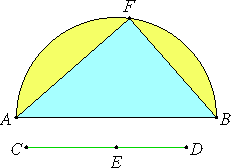# Proposition 30

To find two rational straight lines commensurable in square only such that the square on the greater is greater than the square on the less by the square on a straight line incommensurable in length with the greater.

Set out a rational straight line AB, and two square numbers CE and ED such that their sum CD is not square. Describe the semicircle AFB on AB. Let it be contrived that DC is to CE as the square on BA is to the square on AF, and join FB.

Then, in a similar manner to the preceding, we can prove that BA and AF are rational straight lines commensurable in square only.Since DC is to CE as the square on BA is to the square on AF, therefore, in conversion, CD is to DE as the square on AB is to the square on BF.

X.9

But CD does not have to DE the ratio which a square number has to a square number, therefore neither has the square on AB to the square on BF the ratio which a square number has to a square number. Therefore AB is incommensurable in length with BF.

I.47

And the square on AB is greater than the square on AF by the square on FB incommensurable with AB.

Therefore AB and AF are rational straight lines commensurable in square only, and the square on AB is greater than the square on AF by the square on FB incommensurable in length with AB.

Q.E.D.

## Guide

With the same construction as in the previous proposition, just note at the end that two the lines AB and AF are incommensurable.

This proposition is used in the next three propositions.